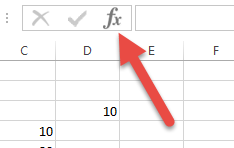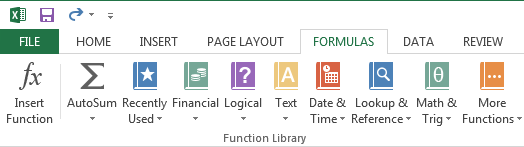# 12 Excel Formulas Every Architect Should Know

Apr 28, 2015Excel is more than just digital graph paper. It’s a serious tool for analyzing and computing data. In order to access this power, however, you need to understand formulas.

This post is part of ArchSmarter’s Excel for Architects series. You can find all of the previous posts in the series here.

If you’re like me, you started using Excel as a way to create nice-looking tables of data – things like building programs or drawing lists. Lots of text and some numbers. Nothing too crazy. If I was feeling a little bold, I’d add a simple formula to add or subtract some cells. That’s about it.

I knew I was using only about 10% of the software but I wasn’t sure what else it could do or how I could access the other functions. I’d heard about formulas but they seemed really confusing. Plus, I was an architect, not a bean counter.

It wasn’t until I ran into a number problem that I realized the true power of Excel. I needed to analyze the leasable area for a large mixed-use project I was working on. We were getting different area numbers from the developers. Since no one likes losing area, I had to dig through the data to figure out what was going on.

So I rolled up my sleeves, took a deep breath, and plunged into the world of Excel formulas. A few hours later, I had a lean and mean spreadsheet that accurately calculated the leasable area. Using the formulas I had built, we could quickly play out scenarios for our client. This spreadsheet ended up being a really useful tool during the design phase.

### Get started with Excel formulas

Inserting a formula into a cell is really easy. Just type an equals sign (=) followed by the formula. You can click the formula icon to open the “Insert Function” dialog.You can also access all of the Excel functions through the “Formulas” ribbon. All of the formulas are grouped into categories. Click the category you want then select the formula from the list. This will open a dialog where you can input the formula parameters.What’s the best way to learn Excel formulas? I’m a big believer in learning by doing. Take a spreadsheet you’ve created and see how you can make it better with formulas. Not sure which ones to try? Here’s my list of 12 Excel formulas every architect should know:

### 1. SUM

Adds together all the values in the specified range. The range can be a single column or multiple columns. You can even specify individual cells by using a comma to separate the values.

=SUM(A5:A25)

### 2. IF

Returns one value if a condition is true and another if the condition is false. Useful for getting a quick overview of your data. You can also use AND or OR within the IF statement to build complex logic.

=IF(A2>B2, “NEED AREA”, “AREA OK”)

### 3. SUMIF

Performs the SUM function only on instances that meet certain criteria. Use SUMIFS to specify multiple criteria.

=SUMIF(A1:A7, “>0”)

=SUMIFS(A1:A7, A1:A7, “>100”, A1:A7, “<200”)

### 4. COUNT, COUNTA, COUNTBLANK

Counts the number of items in the specified range. COUNT only counts numbers, not text or blank cells. COUNTA counts cells that are not empty. This includes number, text and other types of data. COUNTBLANK counts only cells that are blank.

=COUNT(A5:A25)

=COUNTA(A5:A25)

=COUNTBLANK(A5:A25)

### 5. COUNTIF

Similar to COUNT but will count only instances that meet the specified criteria. Use COUNTIFS to specify multiple criteria. For instance, rooms that are greater than 200 SF but less than 500 SF.

=COUNTIF(A1:A8, “>100”)

=COUNTIFS(A1:A8, “>100”, A1:A8, “<200”)

### 6. AVERAGE

Returns the average or arithmetic mean of the specified range of cells.

=AVERAGE(A5:A25)

### 7. MIN

Returns the smallest number in the specified range of cells. This might be useful for finding the smallest area in a programming spreadsheet.

=MIN(A5:A25)

### 8. MAX

Similar to MIN but this formula returns the largest number in a range of cells.

=MAX(A5:A25)

### 9. VLOOKUP

VLOOKUP helps Excel function more like a database than just a spreadsheet application. With it, you can search for values based on other values, which can be located in another part of the worksheet or in a completely different worksheet. In the formula, you need to specify the key value, the range of values you want to search, and the column number of the value you want to return. VLOOKUP is a little tricky to use so I highly recommend checking out this step-by-step guide.

=VLOOKUP(B3,\$A\$17:\$B\$20,2)

### 10. ROUND

Rounds a number to a specified number of digits. Can also use ROUNDUP and ROUNDDOWN to specify the direction of rounding.

=ROUND(7.86, 1) results in 7.9

=ROUNDUP(7.23, 0) results in 8

=ROUNDDOWN(8.85, 1) results in 8.8

### 11. FLOOR and CEILING

These two functions round a number up (CEILING) or down (FLOOR) to the nearest specified multiple.  Useful when rounding currency.

=FLOOR(A1, 10)

=CEILING(A2, 0.25)

### 12. CONCATENATE

Use the CONCATENATE function to join two cells together. This function is great for piecing together text that resides in separate columns. You can also use an ampersand (&) instead of typing out CONCATENATE.

=CONCATENATE(B1, “, “, A1)

=A3& ” ” & B3

### A few more things about formulas

Named ranges are great to use with formulas. Rather than typing the cell range (like A3:B4), you can enter the name (like “Level1Area”). Plus, if the range changes, just update it once in the “Name Manager”. You don’t need to update each formula.

You can review all the available formulas by going to the “Formulas” ribbon and clicking one of the icons in the “Function Library” section. All of the formulas are organized by category. Likewise, you can click the “Insert Function” button to see all the available functions.

You can include one formula in another formula. This is known as a “nesting function”. In Excel 2013, you can nest up to 64 functions.

Copying and pasting formulas can sometimes be tricky. By default, Excel will increment the cell ranges when you paste a formula. Sometimes this is useful, particularly if you’re using SUM to add up a row of values. However, sometimes you want to calculate specific cells. In order to do this, use a “\$” before the cell to designate it as an absolute reference. For example, if I want to multiply cell B4 with cell D3, I would type my formula as “=B4*D3”. Now, if I want to copy this formula down the column but I still want to multiply by cell D3, I would type the formula at “=B4*\$D\$3”. This designates cell D3 as an absolute reference so Excel doesn’t increment it.